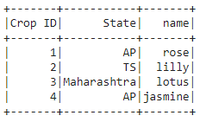Related Articles

# How to convert list of dictionaries into Pyspark DataFrame ?

• Last Updated : 30 May, 2021

In this article, we are going to discuss the creation of the Pyspark dataframe from the list of dictionaries.

We are going to create a dataframe in PySpark using a list of dictionaries with the help createDataFrame() method. The data attribute takes the list of dictionaries and columns attribute takes the list of names.

dataframe = spark.createDataFrame(data, columns)

Example 1:

## Python3

 `# importing module``import` `pyspark`` ` `# importing sparksession from``# pyspark.sql module``from` `pyspark.sql ``import` `SparkSession`` ` `# creating sparksession and giving``# an app name``spark ``=` `SparkSession.builder.appName(``'sparkdf'``).getOrCreate()`` ` `# list of dictionaries of students  data``data ``=` `[{``"Student ID"``: ``1``, ``"Student name"``: ``"sravan"``},``        ``{``"Student ID"``: ``2``, ``"Student name"``: ``"Jyothika"``},``        ``{``"Student ID"``: ``3``, ``"Student name"``: ``"deepika"``},``        ``{``"Student ID"``: ``4``, ``"Student name"``: ``"harsha"``}]`` ` `# creating a dataframe``dataframe ``=` `spark.createDataFrame(data)`` ` `# display dataframe``dataframe.show()`

Output:Example 2:

## Python3

 `# importing module``import` `pyspark`` ` `# importing sparksession from ``# pyspark.sql module``from` `pyspark.sql ``import` `SparkSession`` ` `# creating sparksession and giving ``# an app name``spark ``=` `SparkSession.builder.appName(``'sparkdf'``).getOrCreate()`` ` `# list of dictionaries of crop  data``data ``=` `[{``"Crop ID"``: ``1``, ``"name"``: ``"rose"``, ``"State"``: ``"AP"``},``        ``{``"Crop ID"``: ``2``, ``"name"``: ``"lilly"``, ``"State"``: ``"TS"``},``        ``{``"Crop ID"``: ``3``, ``"name"``: ``"lotus"``, ``"State"``: ``"Maharashtra"``},``        ``{``"Crop ID"``: ``4``, ``"name"``: ``"jasmine"``, ``"State"``: ``"AP"``}]`` ` `# creating a dataframe``dataframe ``=` `spark.createDataFrame(data)`` ` `# display dataframe``dataframe.show()`

Output:Example 3:

## Python3

 `# importing module``import` `pyspark`` ` `# importing sparksession from ``# pyspark.sql module``from` `pyspark.sql ``import` `SparkSession`` ` `# creating sparksession and giving ``# an app name``spark ``=` `SparkSession.builder.appName(``'sparkdf'``).getOrCreate()`` ` `# list of dictionaries of crop  data``data ``=` `[{``"Crop ID"``: ``1``, ``"name"``: ``"rose"``, ``"State"``: ``"AP"``},``        ``{``"Crop ID"``: ``2``, ``"name"``: ``"lilly"``, ``"State"``: ``"TS"``},``        ``{``"Crop ID"``: ``3``, ``"name"``: ``"lotus"``, ``"State"``: ``"Maharashtra"``},``        ``{``"Crop ID"``: ``4``, ``"name"``: ``"jasmine"``, ``"State"``: ``"AP"``}]`` ` `# creating a dataframe``dataframe ``=` `spark.createDataFrame(data)`` ` `# display dataframe count``dataframe.count()`

Output:

`4`

Attention geek! Strengthen your foundations with the Python Programming Foundation Course and learn the basics.

To begin with, your interview preparations Enhance your Data Structures concepts with the Python DS Course. And to begin with your Machine Learning Journey, join the Machine Learning – Basic Level Course

My Personal Notes arrow_drop_up